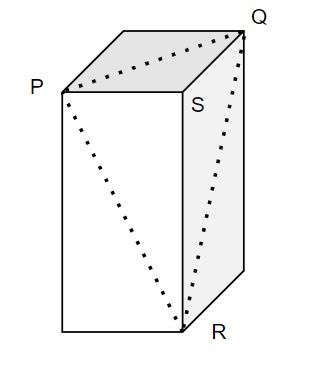# Python Program to find out how many cubes are cut

Suppose, there are several cubes of dimensions a, b, and c, and using them a new box of dimension axbxc is created. a, b, and c are pairwise co-prime; gcd(a, b) = gcd(b,c) = gcd(c, d) = 1. We have to cut the box into two pieces with a single slice as shown in the picture. We have to find out if the box is cut this way, how many cubes are cut into two pieces. We are provided an array that contains the possible three dimensions, and we have to find out our answer from that.The cut is done in this way that it is plane going through the vertices P, Q, and R.

So, if the input is like n = 3, input_array = [[1, 2, 3], [4, 2, 5], [6, 8, 2]], then the output will be [5, 18, 37]

There are 3 different instances given and we have to find out the number of cubes cut. If the cubes are cut in the way shown in the picture; 5, 16, and 37 cubes are cut respectively.

To solve this, we will follow these steps &miuns;

• output := a new list
• for i in range 0 to n, do
• a := input_array[i, 0]
• b := input_array[i, 1]
• c := input_array[i, 2]
• val := floor value of (a * b + a * c + b * c - 1) / 2 mod 1000000007
• insert val at the end of output
• return output

## Example

Let us see the following implementation to get better understanding −

Live Demo

from math import ceil
def solve(n, input_array):
output = []
for i in range(n):
a, b, c = input_array[i], input_array[i], input_array[i]
val = ((a * b + a * c + b * c - 1) // 2 % 1000000007)
output.append(val)
return output
print(solve(3, [[1, 2, 3], [4, 2, 5], [6, 8, 2]]))

## Input

3, [[1, 2, 3], [4, 2, 5], [6, 8, 2]]

## Output

[5, 18, 37]